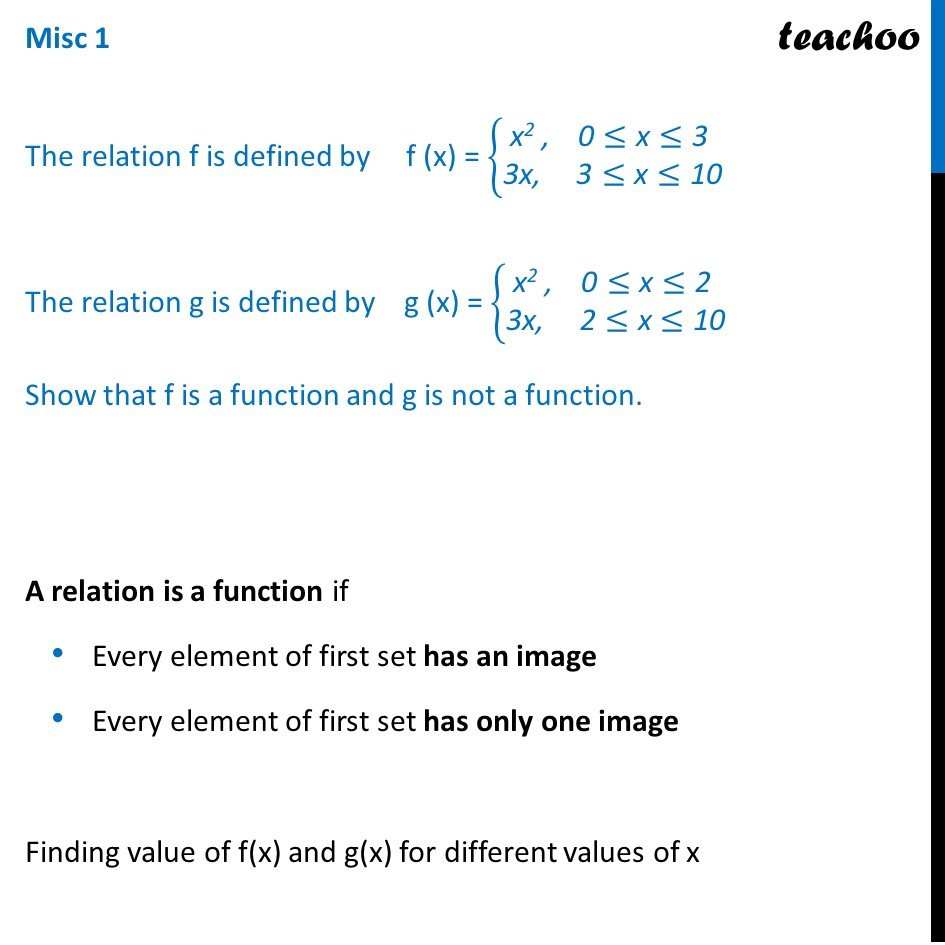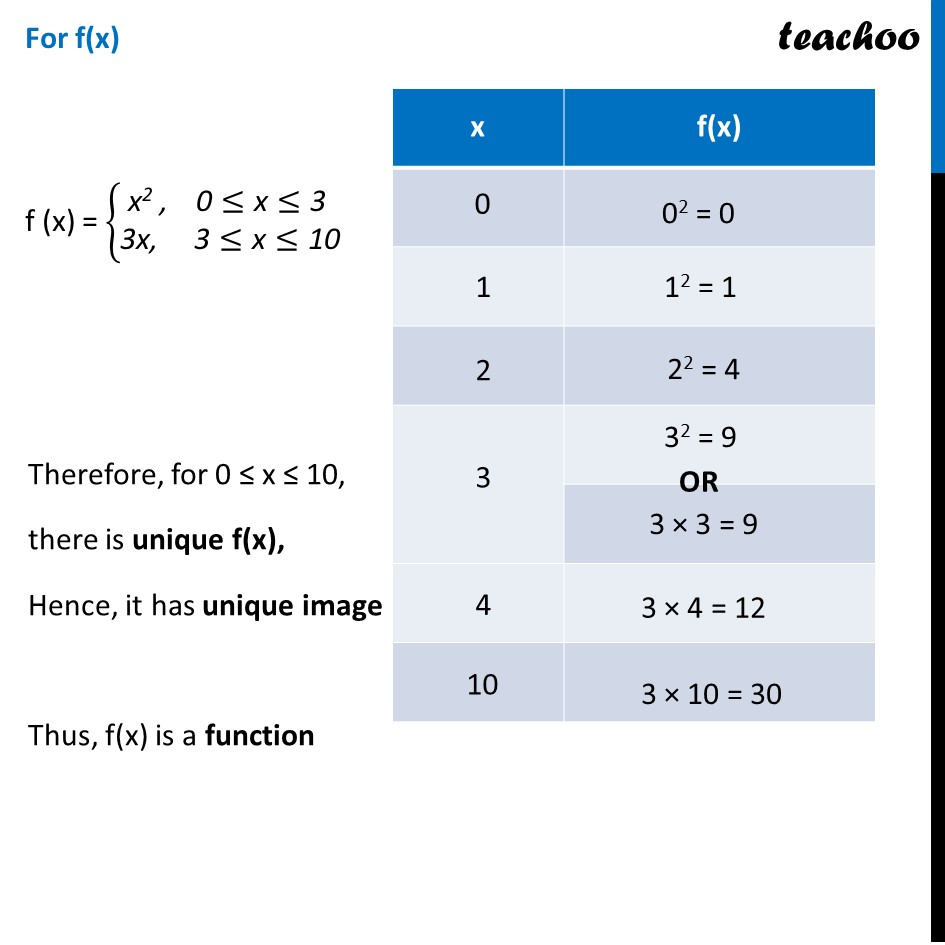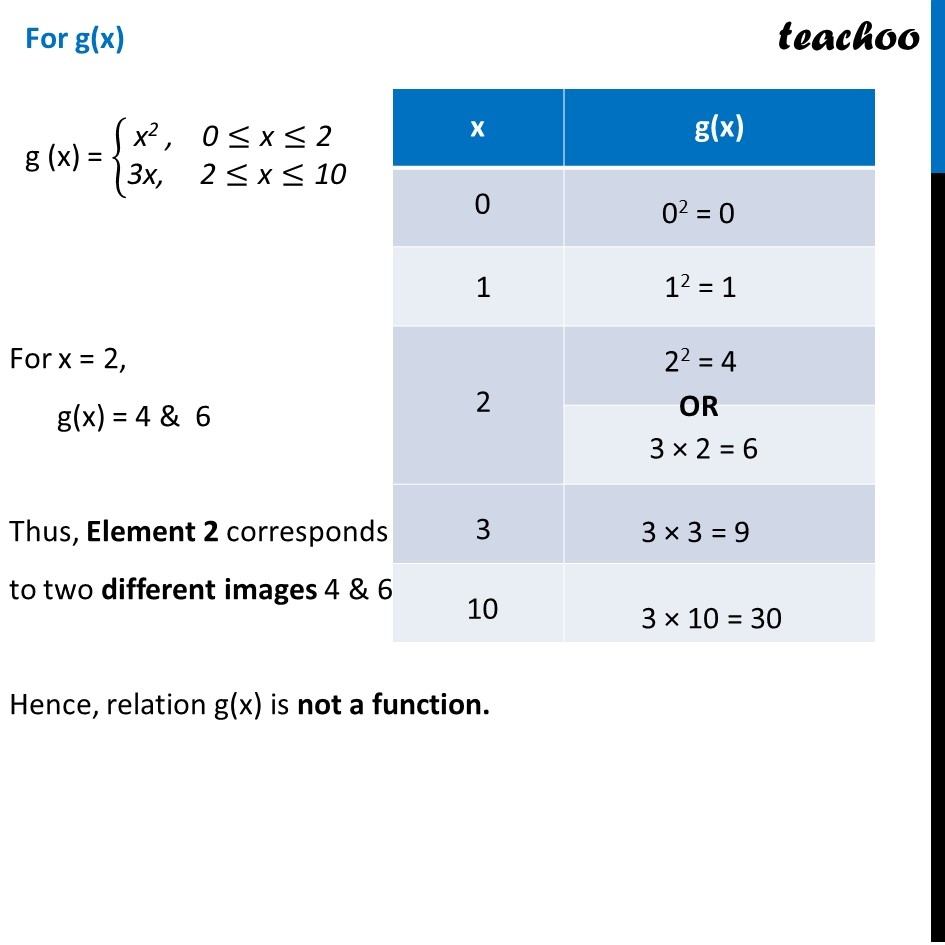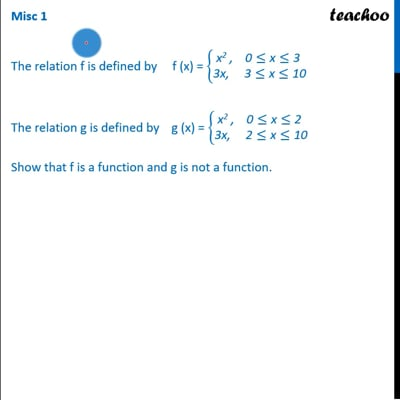Miscellaneous

Chapter 2 Class 11 Relations and Functions (Term 1)
Serial order wiseThis video is only available for Teachoo black users

### Transcript

Misc 1 The relation f is defined by f (x) = {█("x2 , 0" ≤"x" ≤"3 " @"3x, 3" ≤"x" ≤"10" )┤ The relation g is defined by g (x) = {█("x2 , 0" ≤"x" ≤"2 " @"3x, 2" ≤"x" ≤"10" )┤ Show that f is a function and g is not a function. A relation is a function if Every element of first set has an image Every element of first set has only one image Finding value of f(x) and g(x) for different values of x For f(x) f (x) = {█("x2 , 0" ≤"x" ≤"3 " @"3x, 3" ≤"x" ≤"10" )┤ Therefore, for 0 ≤ x ≤ 10, there is unique f(x), Hence, it has unique image Thus, f(x) is a function For g(x) g (x) = {█("x2 , 0" ≤"x" ≤"2 " @"3x, 2" ≤"x" ≤"10" )┤ For x = 2, g(x) = 4 & 6 Thus, Element 2 corresponds “ to two different images 4 & 6. Hence, relation g(x) is not a function.